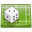# Pre-Kindergarten Math Games

Math Games For Preschool or Pre-Kindergarten on topics like: Addition, Counting objects, Fractions, Math vocabulary, telling time, Geometry & Shapes, Subtraction, Numbers and moreCounting from 1 to 10 and addition game - Penalty shoot game

###Basket ball Counting gamesAddition memory game for Pre - K and KindergartenAddition game for preschool - Audio game adding numbers - matching answersAddition snakes and ladders game for Pre-K and KindergartenSnakes and ladders addition gameShapes - Triangle , Pentagon, Hexagon, Square ...... memory gameGeometry- Shapes: forming a Circle (sphere) puzzle gameGeometry - shapes: create a hexagon from the puzzleGeometry- Shapes : create a square from the squares in the puzzleCounting from 1 to 10 and addition game- Walk the plank gameSubtractions Game- En garde Duel GameAddition Game - Basketball gameAddition Game -Penalty shoot gameAddition Game- Fling the teacher gameNumber rearrangement Game- Arrange in order- walk the plank gameComparisons Game- En Garde Duel GameGeometry Game- Fing the teacher gameMissing Number Game -Penalty shoot gameSubtraction Game 1 -10- En gard duel gameSubtraction Game - Basketball gameSubtraction Game - Fling the teacherTelling the time game - memory gameNumber Spelling Game - Memory GameNumber Spelling Game - Walk the plank gameAlgebra is fun. These games will help kids practice algebra in a fun way. Kids relate very well to games . Several algebra topics are covered in the form of interactive games and include the following:

Algebra Worksheets & PrintablesThese worksheets are printable PDF exercises of the highest quality. Writing reinforces Maths learnt. These worksheets contain pre-algebra & Algebra exercises suitable for preschool, kindergarten, first grade to eigth graders, 6th grade math worksheets . The following algebra topics are covered among others:This section contains worked examples of problems and vital algebra formulae that are needed through out the theme. It is very important to master using these formulae though some times presenting them in the most simplified manner is necessary for young learners. This section is a step-by-step presentation of how to use algebra formulae on all the topics covered in this site which include formulae on -linear equations, inequalities, decimals, fractions, exponents, graphing linear equations, binomial theorem, pythagoras theorem, quadratic equations, algebraic expressions, factorization, ratios, geometry, integers, order operations, angles, simple equations, slope, arithmetic progression, LCM & HCF, coefficients, quadratic equations, square roots & more-

These quizzes range from multiple choice algebra quizzes, gap fill quizzes, matching exercises, hotspot quizzes with graphics and more for interactive algebra & pre-algebra practice. - for first grade, second grade, third grade, fourth grade, fifth grade, sixth grade and eight grade.

Web site General Content:

This site is all about the following: Algebra exercises for children, Algebra & pre - algebra for kids, children, Algebra activities Online for children, Algebra games for kids, elementary algebra, first grade algebra, algebra games, worksheets, printables, exercises, free online exercises, free algebra downloads, printable PDF,linear equations, inequalities, decimals, fractions, exponents, graphing linear equations, binomial theorem, pythagoras theorem, quadratic equations, algebraic expressions, factorization, ratios, geometry, integers, order operations, angles, simple equations, slope, arithmetic progression, LCM & HCF, coefficients quadratic equations, square roots & more-

math, printable Algebra exercises, Algebra worksheets, Pre -algebra worksheets, pre-algebra, , Algebra for children, Algebra topics, Algebraic processes, algebra for kids, worksheets, printables on algebra, linear equations, polynomials, introduction to algebra, pythagoras theorem, fractions, algebra online, algebra is fun, algebra printable games, algebra formulae & more